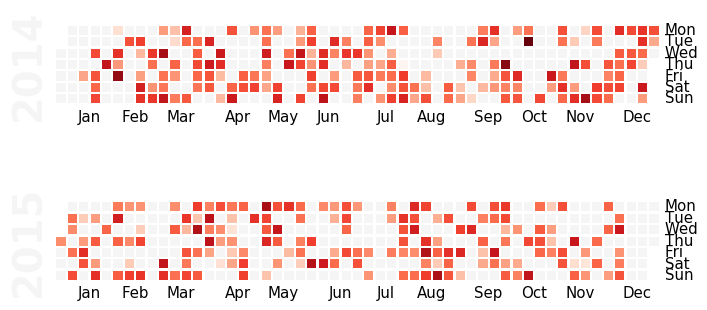# Calendar heatmaps from Pandas time series data¶

Plot Pandas time series data sampled by day in a heatmap per calendar year, similar to GitHub’s contributions plot, using matplotlib.

## Usage¶

Assume we have some weighted events as a Pandas Series with a DatetimeIndex. They could be Git commits (with the diff size as weight), mileage of your runs, or minutes spent on telemarketing phone calls driving you crazy.

For illustration purposes we just create 500 events as random float values assigned to random days over a 700-day period:

```import numpy as np; np.random.seed(sum(map(ord, 'calmap')))
import pandas as pd
import calmap

all_days = pd.date_range('1/15/2014', periods=700, freq='D')
days = np.random.choice(all_days, 500)
events = pd.Series(np.random.randn(len(days)), index=days)
```

Using `yearplot()`, we can easily plot a heatmap of these events over a year:

```calmap.yearplot(events, year=2015)
```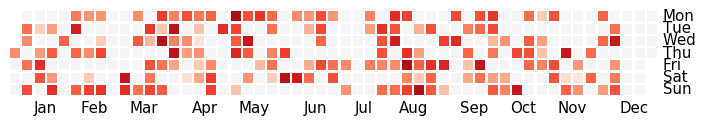Or we can use `calendarplot()` to plot all years as subplots into one figure:

```calmap.calendarplot(events, monthticks=3, daylabels='MTWTFSS',
dayticks=[0, 2, 4, 6], cmap='YlGn',
fillcolor='grey', linewidth=0,
fig_kws=dict(figsize=(8, 4)))
```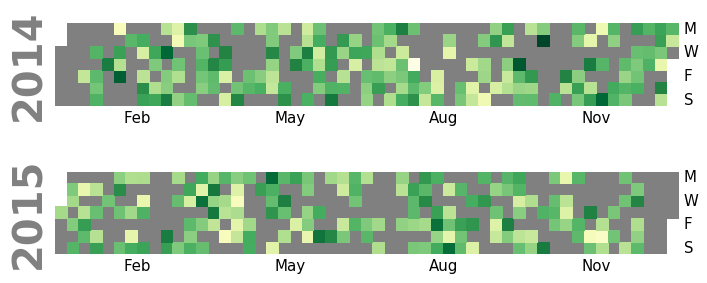## Installation¶

To install the latest release via PyPI using pip:

```pip install calmap
```

The latest development version can be found on GitHub.

## API documentation¶

`calmap.``yearplot`(data, year=None, how=u'sum', vmin=None, vmax=None, cmap=u'Reds', fillcolor=u'whitesmoke', linewidth=1, linecolor=None, daylabels=['Mon', 'Tue', 'Wed', 'Thu', 'Fri', 'Sat', 'Sun'], dayticks=True, monthlabels=['Jan', 'Feb', 'Mar', 'Apr', 'May', 'Jun', 'Jul', 'Aug', 'Sep', 'Oct', 'Nov', 'Dec'], monthticks=True, ax=None, **kwargs)[source]

Plot one year from a timeseries as a calendar heatmap.

Parameters: data : Series Data for the plot. Must be indexed by a DatetimeIndex. year : integer Only data indexed by this year will be plotted. If None, the first year for which there is data will be plotted. how : string Method for resampling data by day. If None, assume data is already sampled by day and don’t resample. Otherwise, this is passed to Pandas Series.resample. vmin, vmax : floats Values to anchor the colormap. If None, min and max are used after resampling data by day. cmap : matplotlib colormap name or object The mapping from data values to color space. fillcolor : matplotlib color Color to use for days without data. linewidth : float Width of the lines that will divide each day. linecolor : color Color of the lines that will divide each day. If None, the axes background color is used, or ‘white’ if it is transparent. daylabels : list Strings to use as labels for days, must be of length 7. dayticks : list or int or bool If True, label all days. If False, don’t label days. If a list, only label days with these indices. If an integer, label every n day. monthlabels : list Strings to use as labels for months, must be of length 12. monthticks : list or int or bool If True, label all months. If False, don’t label months. If a list, only label months with these indices. If an integer, label every n month. ax : matplotlib Axes Axes in which to draw the plot, otherwise use the currently-active Axes. kwargs : other keyword arguments All other keyword arguments are passed to matplotlib ax.pcolormesh. ax : matplotlib Axes Axes object with the calendar heatmap.

Examples

By default, yearplot plots the first year and sums the values per day:

```calmap.yearplot(events)
```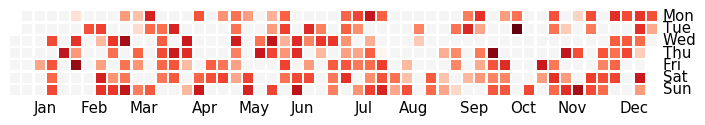We can choose which year is plotted with the year keyword argment:

```calmap.yearplot(events, year=2015)
```The appearance can be changed by using another colormap. Here we also use a darker fill color for days without data and remove the lines:

```calmap.yearplot(events, cmap='YlGn', fillcolor='grey',
linewidth=0)
```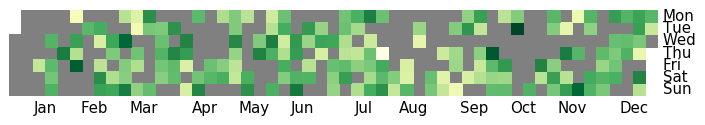The axis tick labels can look a bit crowded. We can ask to draw only every nth label, or explicitely supply the label indices. The labels themselves can also be customized:

```calmap.yearplot(events, monthticks=3, daylabels='MTWTFSS',
dayticks=[0, 2, 4, 6])
```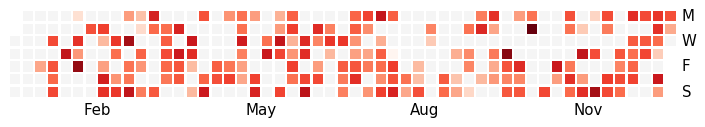`calmap.``calendarplot`(data, how=u'sum', yearlabels=True, yearascending=True, yearlabel_kws=None, subplot_kws=None, gridspec_kws=None, fig_kws=None, **kwargs)[source]

Plot a timeseries as a calendar heatmap.

Parameters: data : Series Data for the plot. Must be indexed by a DatetimeIndex. how : string Method for resampling data by day. If None, assume data is already sampled by day and don’t resample. Otherwise, this is passed to Pandas Series.resample. yearlabels : bool Whether or not to draw the year for each subplot. yearascending : bool Sort the calendar in ascending or descending order. yearlabel_kws : dict Keyword arguments passed to the matplotlib set_ylabel call which is used to draw the year for each subplot. subplot_kws : dict Keyword arguments passed to the matplotlib add_subplot call used to create each subplot. gridspec_kws : dict Keyword arguments passed to the matplotlib GridSpec constructor used to create the grid the subplots are placed on. fig_kws : dict Keyword arguments passed to the matplotlib figure call. kwargs : other keyword arguments All other keyword arguments are passed to yearplot. fig, axes : matplotlib Figure and Axes Tuple where fig is the matplotlib Figure object axes is an array of matplotlib Axes objects with the calendar heatmaps, one per year.

Examples

With calendarplot we can plot several years in one figure:

```calmap.calendarplot(events)
```